• Shuffle
Toggle On
Toggle Off
• Alphabetize
Toggle On
Toggle Off
• Front First
Toggle On
Toggle Off
• Both Sides
Toggle On
Toggle Off
Toggle On
Toggle Off
Front

## Card Range To Study

throughPlay buttonPlay buttonProgress

1/160

Click to flip

Use LEFT and RIGHT arrow keys to navigate between flashcards;

Use UP and DOWN arrow keys to flip the card;

H to show hint;

 Rate Graphs and OrdersDefine Rate of reaction The change in concentration of a reactant or product per unit time Rate Graphs and Ordersrate = ? units ? change in concentration of reactant or product / time for the change to take place units = mol dm-3/s = mol dm-3 s-1 Rate Graphs and OrdersHow is a concentration represented? Square brackets[CO2] = concentration of CO2 Rate Graphs and OrdersDescribe what happens to the concentration of reactants as the reaction progresses The concentrations of the reactants decrease because fewer collisions take place per second between reactants and the rate slows down. Rate Graphs and OrdersHow is rate represented? In a concentration-time graph : the rate is equal to the slope (gradient) of the curve Rate Graphs and OrdersDraw a concentration-time graph over the course of a reaction and draw a tangent for the initial rate and a tangent as the reaction proceeds.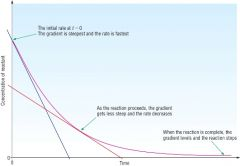Rate Graphs and OrdersDefine the initial rate of reaction The change in concentration of a reactant, or product, per until time at the start of the reaction when t = 0 Rate Graphs and Orders Draw a concentration-time graph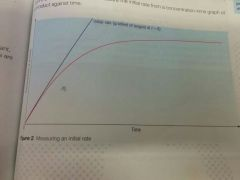Rate Graphs and OrdersHow can you monitor the reaction rate of reactions involving acids or bases? measure: -pH changes by carrying out titrations- pH changes by using a pH meter Rate Graphs and OrdersHow can you monitor the reaction rate of reactions that produce gases? measure: -the change in volume or pressure-the loss in mass of reactants Rate Graphs and OrdersHow can you monitor the reaction rate of reactions that produce visual changes? observe: -the formation of a precipitate-a colour change Rate Graphs and Orders How does a colorimeter work? Measures the intensity of different colours in a liquid - the colourimeter passes filtered light through the sample and the intensity of the colour is recorded. Rate Graphs and OrdersWhat is an example of a reaction that produces a colour change? Reaction of Bromine and Methanoic acid. Br2 (aq) + HCOOH (aq) -----> 2Br- (aq) + 2H+ (aq) + CO2 (g) The orange colour of the Bromine becomes less intense as its concentration falls. The intensity of the colour depends on the concentration of Bromine, so the reaction rate can be followed by colorimetry. Rate Graphs and OrdersHow to calculate gradient ∆Y / ∆ X Rate Graphs and Orders What effect does concentration have on a reaction? The greater the concentration -the larger the number of collisions per second-the faster the reaction Rate Graphs and Orders For a reactant, A, what is the relationship with the rate Rate ∝ [A]^x Rate is proportional to the concentration of reactant A x = the order with respect to A Rate Graphs and Orders Describe Zero order If the order is 0 with respect to reactant A, then Rate ∝ [A]^0The rate is unaffected by changing the concentration of A (anything to the power of 0 = 1) Rate Graphs and Orders Describe First order If the order is 1 with respect to a reactant B then Rate ∝ [B]^1If [B] increase x2 then the rate also increases x2If [B] increases x 3 then the rate also increases x3 Rate Graphs and Orders Describe second order If the order is 2 with respect to a reactant C then Rate ∝ [C]^2 If [C] increase by 2, the rate increases by 2^2 = 4If [C] increases by 3 the rate increases by 3^2 = 9 times Rate Graphs and Orders What is the rate equation? What is the overall order? A + B --> Cthen rate = k[A]^m [B] ^n Overall order = m+n so for k[A]^1[B]^2 = overall order 3 Rate Graphs and Orders What is the rate constant ? k, the constant that links the rate of reaction with the concentrations of the reactants raised to the powers of their orders in the rate equation. Rate Graphs and Orders How can the rate equation be determined? Only from experimental results. The orders are not equal to the balancing numbers in the overall equation! Rate Graphs and Orders What do the units of k depend on? The overall order of the rate equation Rate Graphs and Orders Calculate the units of K for a zero order reaction rate = k[A] 0 = k units of k = mol dm-3 s-1 Rate Graphs and Orders Calculate the units of k for a first order reaction rate = k[A]^1 = k[A] rearrange to givek = rate/[A] mol dm-3 s-1/ moldm-3 (mol dm-3's cancel) leaves s-1 Rate Graphs and Orders Calculate the units of k for a second order reaction rate = k[A]^2k= rate/[A]^2mol dm-3 s-1 / (moldm-3)(mol dm-3) mol dm-3 cancels-1/mol dm-3 = dm3 mol-1 s-1 (when you bring the bottom units up to the top, the signs become reversed?) Rate Graphs and Orders Calculate the units of k for a third order reaction rate = k[A]^2[B]k = rate/[A]^2[B]mol dm-3 s-1 / (mol dm-3)^2 (mol dm-3) mol dm-3's cancel leavings-1/ (mol dm-3) ^2 s-1 / mol^2 dm-6(when they come to the top the signs are reverse) dm^6 mol^-2 s-1 Rate Graphs and Orders Worked example:The reaction between H2 and NO has the following rate equation:rate = k[H2][NO]^26 x 10^-3 moldm-3 H2 was reacted with 3 x 10^-3 moldm-3 NO. Initial rate = 4.5 x 10^-3 mol dm-3 s-1. Calculate the rate constant, k, and its units.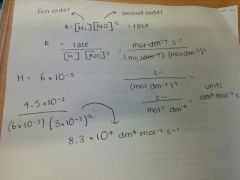Rate Graphs and OrdersDefine Half-life The half-life of a reactant is the time taken for the concentration of the reactant to reduce by half Rate Graphs and Orders What is the half-life of a first order graph? Always costant Rate Graphs and Orders How does half-life change with concentration of a first order reaction? It does not change, it stays the same. The half-life of a first order reaction is independent of the concentration Rate Graphs and Orders Draw the shapes of the concentration time graphs of Zero order, first order and second order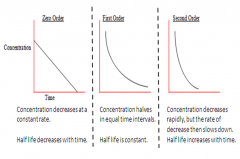Rate Graphs and Orders How else can you determine the order with respect to a reactant? By plotting a rate-concentration graph Rate Graphs and Orders Draw rate-concentration graphs for zero order, first order and second order.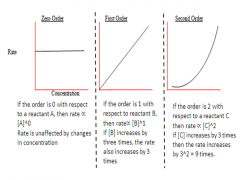Rate Graphs and Orders Other than drawing a tangent to the curve at t=0, how can you calculate initial rate? Measure the time taken for a certain amount of product to be formed. Clock reactions measure the time from the start of the reaction until there is a visual change such as -the appearance of a ppt-the disappearance of a solid-a change in colour. During a clock reaction you measure the time for the initial part of the reaction to take place - the initial rate = 1/t.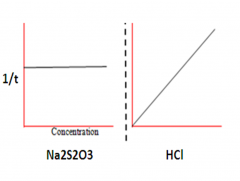Rate Graphs and Orders Na2S2O3 + HCl -> 2NaCl + S + SO2 + H2OThis reaction forms Sulfur as a cloudy ppt. It was timed how long it took for the ppt to form whena)changing the conc of HClb)changing the conc of Na2S2O3rate was calculated 1/t. These concentration time graphs were produced: what order do each reactants have and what is the rate equation? Na2S2O3 = Zero order Rate is proportional to [Na2S2O3]^0 HCl = first order rate proportional to [HCl]^1 Rate = k[HCl]^1[Na2S2O3]^0Rate = k[HCl]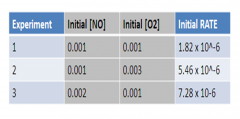Rate Graphs and Orders There is another way to calculate the orders of reactants: you are given information as to how the rate changes with changes in concentrations of different reactants. Work out order from this: 2NO + O2 --> 2NO2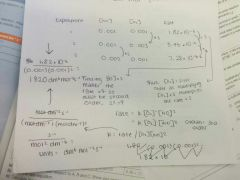Rate Graphs and OrdersThe larger the value of k... The faster the reaction Rate Graphs and OrdersDescribe from AS chemistry how temperature effects molecules An increase in temperature increases the energy of molecules. There are then more frequent collisions and more of the collisions exceed the activation energy of the reaction. Rate Graphs and OrdersHow does temperature increase the rate of reactions in relation to k? Raising the temperature of most reactions by increasing the rate constant, k. Rate determining stepWhat is a reaction mechanism? A series of steps that together make up the overall reaction Rate determining stepDefine the rate determining step The slowest step in the reaction mechanism of a multi step reaction Rate determining stepDescribe why the rate determining step determines the rate The rate determining step is the slowest step and so becomes an obstacle to the whole process -reactants can become products only as fast as they get through this step. The overall reaction can be no faster than the slowest step. Rate determining step What is the correlation between the rate equation and the rate determining step? The reactants that appear in the rate equation are the reactants involved into the rate determining step.The order with respect to the reactant tells you how many particles of the reactant are involved in the rate determining step EquilibriumWhen is dynamic equilibrium established? In a closed system when the rate of the forward reaction is the same as the rate of the reverse reaction, and the concentrations of the reactants and the products remain the same. EquilibriumWhat is the Equilibrium law? A law that tells us the relative proportions of reactants and products present at equilibrium. It states that for the equilibrium aA + bB ↔ cC + dD (where lower case letters are the balancing numbers) then Kc = [C]^c[D]^d / [A]^a[B]^b EquilibriumExplain what each parts of this mean Kc = [C]^c[D]^d / [A]^a[B]^b Kc = the equilibrium constant in terms of concentrations [products]^balancing numbers / [reactants]^balancing numbers of overall reaction EquilibriumDescribe what happens to the concentrations of reactants and products as a reaction proceeds, and use a graph to show how equilibrium is achieved.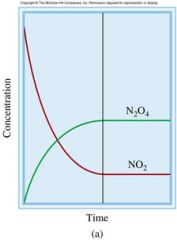-At the beginning of the reaction the concentration of reactants is high, and the concentrations of products are low so the reverse reaction can happen only very slowly. -As the reaction proceeds there are fewer reactants to form products, the concentration decreases so the rate of the forward reaction decreases-There are more product molecules, the rate of the reverse reaction increases to increase the concentration of reactants. Eventually a dynamic equilibrium is established where the forward reaction takes place at exactly the same rate as the reverse reaction. The concentrations of the reactants and the products are constant. EquilibriumWhat is the equation for Kc for the following reaction? H2 + I2 ↔ 2HI Kc = [HI]^2 / [H2][I2] Equilibrium What is a) homogeneous equilibrium? b) heterogeneous equilibrium? a) an equilibrium in which all the species making up the reactants and products are in the same physical state b) an equilibrium in which in which species making up the reactants and products are in different physical states Equilibrium What are the units of Kc? The units of Kc varies for each equilibria. Equilibrium Work out the units for Kc = [HI]^2 / [H2][I2] ( Mol dm-3 )^2 / (mol dm-3)(mol dm-3) all cancels. 0 units. Equilibrium Work out the units for Kc = [SO3]^2 / [SO2]^2[O2] (mol dm-3)^2 / (moldm-3)^2 (moldm-3) = mol-1dm3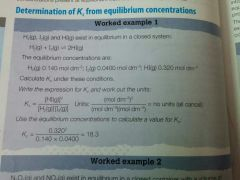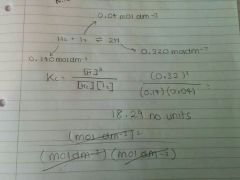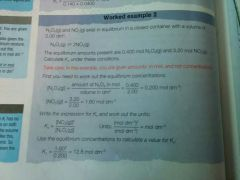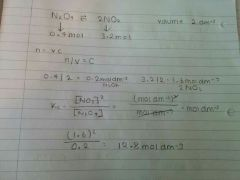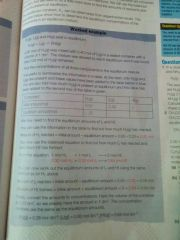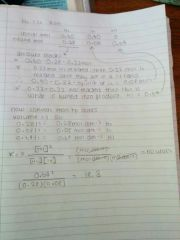EquilibriumWhat does Kc indicate? The extent of a chemical reaction EquilibriumWhat does a Kc value of 1 indicate? The position of equilibrium is halfway between reactants and products. EquilibriumWhen Kc > 1? the reaction is product-favouredThe products on the right hand side predominate at equilibrium EquilibriumWhen Kc < 1 The reaction is reactant-favoured The reactants on the left hand side predominate at equilibrium. Equilibrium(from AS) what does an increase in temperature do to the position of equilibrium? Shifts the position of equilibrium to the endothermic direction (^H +ve) Equilibrium(from AS) what does a decrease in temperature do to the position of equilibrium? shifts the position of equilibrium to the exothermic direction (^H -ve) EquilibriumWhen does the value of Kc change? ONLY WITH TEMPERATUREEEEE Equilibrium(dis just a rule man)When the forward reaction is endothermic... when temperature increases, Kc increases. The increase in temperature will favour the forward reaction, so the yield of products on RIGHT will increase and yield of reactants decreases. Equilibrium (dis just a rule man) When the forward reaction is exothermic... when temperature increases, Kc decreases. The increase in temperature will favour the reverse reaction, so the yield of products decreases and yield of reactants increases. EquilibriumHow does changing concentration and pressure affect Kc? It doesn't. Kc is unaffected by changes in concentration and pressure. EquilibriumSo what happens when the concentration of a thing increases? The equilibrium must shift to restore the Kc value. EquilibriumKc = [NO2]^2 / [N2O4] = 1.6^2 / 0.2 = 12.8 moldm-3When the concentration of N2O4 increases, it gives a Kc value of 6.4mol dm-3. How will the equilibrium shift to restore the original Kc value? Since you want to increase the Kc value (from 6.4 to 12.8) you need to increase the concentration of NO2 on the top of the fraction and decrease the concentration of N2O4 on the bottom: (more pizza divided by less people = more pizza per person) Equilibrium shifts from left to right. EquilibriumKc = [NO2]^2 / [N2O4] = 12.8 a) When the pressure is doubled, what happens to the concentrations? b) if the new kc value is 25.6, how does the equilibrium shift to restore the original Kc value? a) they also doubleb) Since Kc needs to decrease, you want to decrease pizza size and increase the amount of people who are having pizza - so increase the concentration of N2O4 on the bottom and decrease the concentration of NO2 on the top. Equilibrium shifts from right to left. EquilibriumHow does the presence of a catalyst affect Kc? IT DOESN'T. Catalysts do not affect the position of equilibrium but speed up the rate of the forward and reverse reactions by the same amount. Equilibrium is reached more quickly but its position is unchanged. EquilibriumSummarise the knowledge of Kc. Include: a) What is tells youb) What a large value indicatesc) How temperature affects Kc d) How you write a Kc equilibrium expression a) Kc compares the concentrations of reactants and products present at equilibrium = how farb) Large value = position of equilibrium lies well to the right-hand side, product favouredc) If forward reaction endothermic, Kc increases as temperature increases. If the forward reaction is exothermic, Kc decreases as temperature increases.d) Use the overall balanced equation. EquilibriumSummarise the knowledge of K. Include: a) What it tells youb) What a large value indicatesc) How temperature affects Kd) How you can measure K. a) Measures the rate of a reaction = how fastb) Large value = fast ratec) Increase in temp = increase in kd) Only experimentally EquilibriumFor the equilibrium below, discuss the yields with increasing temperature N2 + 3H2 <-> 2NH3 -92jkmol-1 The forward reaction is exothermic. An increase in temperature would cause the reverse reaction (endothermic) to be favoured, so a low yield of NH3. At high temperatures, Kc is small (because when the forward reaction is exothermic, with temperature increases, Kc decreases), but K is high. At low temperatures, Kc is large (because the reverse reaction is endothermic), but K is small. So, low temp = large amount of ammonia slowlyhigh temp = small amount of ammonia, equilib reached quickly. = compromise pls. Acids, Bases and Buffers What is a Bronsted-Lowry acid? A proton donor Acids, Bases and Buffers What is a Bronsted-Lowry base? A proton acceptor. Acids, Bases and Buffers Acids, Bases and Buffers What is an alkali? A base that dissolves in water to form OH- ions. Acids, Bases and Buffers What happens when an acid is added to water? Use HCl as an example. It dissociates releasing H+ ions into solution.HCl (aq) ---> H+ (aq) + Cl- (aq) Acids, Bases and Buffers Why is HCl a monobasic acid? Each molecule can release 1 proton Acids, Bases and Buffers Draw the two stages of the dissociation of the dibasic acid H2SO4. H2SO4 (aq) ---> H+ (aq) + HSO4 - (aq)HSO4- (aq) <-> H+ +SO4 2- Acids, Bases and Buffers What acid-base reactions do you need to know? Carbonates, bases, alkalis. Acids, Bases and Buffers Define neutralisation A chemical reaction in which an acid and a base react together to produce a salt and water Acids, Bases and Buffers What is the basic word equation for the reaction ofa) acids and solid carbonatesb) acids and basesc) acids and alkalis a) Acid + Carbonate -> salt + CO2 + H2Ob) Acid + Base -> salt + H2Oc) Acid + Alkali -> Salt + H20 Acids, Bases and Buffers Draw the equation, ions and ionic equation for the reaction of HCl and CaCO3 (a solid carbonate) 2HCl (aq) + CaCO3 (s) --> H2O (l), CaCl2 (aq), CO2 (g)2H+ + 2Cl +CaCO3 ---> Ca2+ +2Cl- + CO2 + H2O(Cancel anything that's the same on both sides) 2H+ (aq) + CaCO3 ---> Ca2+ + CO2 + H2O Acids, Bases and Buffers Draw the equation, ions and ionic equation for the reaction of HCl and Na2CO3 (a carbonate in solution)) When a carbonate is in solution, it dissociates, so the ionic equation simplifies. Full: 2HCl (aq) + Na2CO3 (aq) --> 2NaCl (aq) + CO2(g) + H2O (l)Ions: 2H+ + 2Cl- + 2Na+ + CO3 2- ---> CO2 + H2O + 2Na+ + 2CL- (Cancels)2H+ + CO3 2---> CO2 + H2O Acids, Bases and Buffers Draw the full equation, ions and ionic equation for threaction of HNO3 (acid) and MgO (base) Full : 2HNO3 + MgO ---> Mg(NO3)2 + H2O All ions: 2H+ + 2NO3- + MgO ---> Mg2+ + 2NO3- + H2 (Cancel)ionic: 2H+ + MgO ---> Mg 2+ + H2O Acids, Bases and Buffers Draw the full equation, ions and ionic equation for the reaction between H2SO4 + KOH. Full: H2SO4 + 2KOH ---> K2SO4 + 2H2OIons: 2H+ +SO42- + 2K+ + 2OH- ----> 2K+ + SO42- + 2H2OIonic equation: H+ + OH- ---> H2O Acids, Bases and Buffers Acid + Metal ---> ? Salt + H2 Acids, Bases and Buffers Draw the reaction between HCl + Mg. Full equation: 2HCl + Mg ---> MgCl2 + H2 Ions: 2H+ + 2Cl- + Mg ---> Mg 2+ + 2Cl - + H2Ionic equation: 2H+ + Mg ---> Mg 2+ + H2 Acids, Bases and Buffers What must a molecule of an acid contain? Hydrogen that can be released as H+ . Acids, Bases and Buffers What else does an acid require to release a proton? A proton acceptor (a base) Acids, Bases and Buffers Draw the formation of the Hydronium ion as an example of the release of a proton from an acid when added to water. (what accepts the proton?)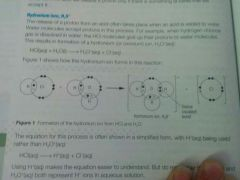HCl (aq) + H2O ---> H3O (aq) + Cl- (aq) Acids, Bases and Buffers Draw the simplified reaction for this reaction. HCl (aq) ---> H+ (aq) + Cl- (aq) Acids, Bases and Buffers Define acid-base pair A pair of two species that transform into each other by gain or loss of a proton Acids, Bases and Buffers Show how HNO2 and NO2- are conjugate acid-base pairs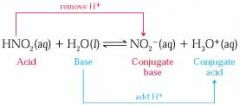Acids, Bases and Buffers Describe what is happening in the following acid-base equilibria: HNO2 + H2O <-> H3O + + NO2 - Forward: Acid HNO2 releases a proton to form its conjugate base, NO2 - Base H2O accepts the proton to form its conjugate acid, H3O+Reverse: Acid H3O+ releases a proton to form its conjugate base H2OBase NO2- accepts the proton to form its conjugate acid, HNO2. Acids, Bases and Buffers pH = ? -log[H+ (aq)] Acids, Bases and Buffers [H+ (aq)] = ? 10 ^-pH Acids, Bases and Buffers Low pH = ? High [H+] Acids, Bases and Buffers High pH = ? Small [H+] Acids, Bases and Buffers A pH change of 1 changes [H+] by? 10x Acids, Bases and Buffers Define strong acid An acid that completely dissociates in solution Acids, Bases and Buffers Define weak acid An acid that partially dissociates in solution. Acids, Bases and Buffers List the 6 most common strong acids. HCl HNO3H2SO4HBrHIHClO4 (perchloric) Acids, Bases and Buffers Describe whether Ethanoic acid is a weak or strong acid. When ethanoic acid dissociates in water, an equilibrium is set up where the equilibrium position lies to the left. CH3COOH + H2O <-> CH3COO- + H3O + There are only small concentrations of H+ and CH3COO- and H+, whereas there is a large conc of CH3COOH. Acids, Bases and Buffers What is the acid dissociation constant? Ka, of an acid HA is defined as: Ka = [H+][A-]/[HA] (concentration of ionic products/concentration of undissociated acid) Acids, Bases and Buffers Units of Ka? ALWAYS mol dm-3 Acids, Bases and Buffers Large Ka value = ? strong acid - large extent of dissociation Acids, Bases and Buffers Small Ka value = weak acid = small extent of dissociation Acids, Bases and Buffers pKa =? -log10Ka Acids, Bases and Buffers Ka = ? 10^-pKa Acids, Bases and Buffers Low value Ka = High value pKa Acids, Bases and Buffers High value Ka = Low value pKa Acids, Bases and Buffers The smaller the value of pKa (thus the bigger the value of Ka) = the stronger the acid. Acids, Bases and Buffers For a strong acid, [H+] = [HA]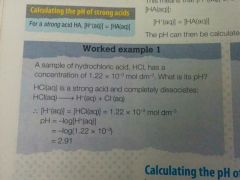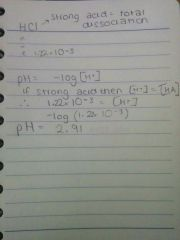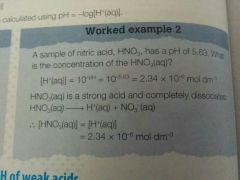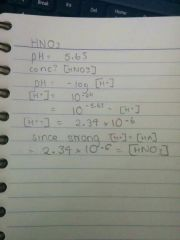Acids, Bases and Buffers What is the Ka equation for weak acids? Ka = [H+]^2 / [HA]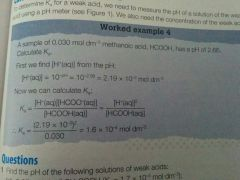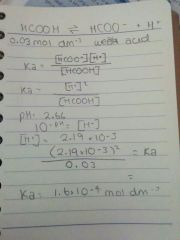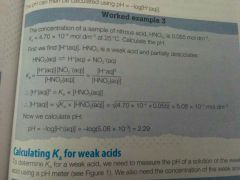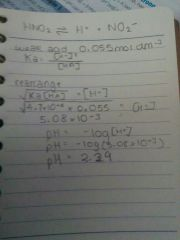Acids, Bases and Buffers Draw an equilibria for the ionisation of water. include the position of equilibrium and equation for Kc. <----------------------- (equilibrium)H2O (l) <-> H+ (aq) + OH- (aq) Remember- Kc = equilibrium constant, Kc = [products]/[reactants] (to the power of their balancing numbers). It is a measure of the proportion of reactants and products present at equilibrium.Since Kc = [OH-][H+]/[H2O] then Kc x [H2O] = [OH-][H+] Acids, Bases and Buffers What is [H2O]? The concentration of water in mol dm-3 which is a CONSTANT VALUE OF 55.6MOL DM-3. Acids, Bases and Buffers What is the ionic product of water? Since Kc and [H2O] are both constants, they are combined to give a new constant, Kw = ionic product of water. If Kc x [H2O] = [OH-][H+] Kw = [OH-][H+]. Acids, Bases and Buffers Define ionic product of water Kw = [H+][OH-]At 25 degrees, Kw = 1.00 x 10^-14 mol 2 dm -6 Acids, Bases and Buffers Function of Kw? Controls the balance between [H+] and [OH-] in all aqueous solutions Acids, Bases and Buffers In water and neutral solutions, what is the relationship between [H+] and [OH-] ? [H+] = [OH-] Acids, Bases and Buffers In acidic solutions, what is the relationship between [H+] and [OH-] [H+] > [OH-] Acids, Bases and Buffers In alkaline solutions, what is the relationship between [H+] and [OH-] ? [H+] < [OH-] Acids, Bases and Buffers If you know that Kw is always 1x10^-14 at 25 degrees, how can you work out the concentrations of H+ or OH-? Because Kw = [H+][OH-] 1x10^-14 = [H+][OH-]. If you know the conc of either H+ or OH-, then you can just divide it by the Kw to get the other value.At 25 degrees [H+] = 10^-7, calculate [OH-] = 1x10^-14 / 10^-7 = 10^-7. (you may also notice that the sum of the indicies adds up to -14.) Acids, Bases and Buffers what is the strength of a base? A measure of its dissociation in solution to generate OH- ions. Acids, Bases and Buffers Draw the dissociation of NaOH and NH3. (one is weak, one is strong) NaOH + aq ----> Na + + OH-Strong. NH3 + H2O <-> NH4+ + OH- weak <---- equilibrium Acids, Bases and Buffers 3 most common strong bases? NaOH + KOH + Ca(OH)2. Acids, Bases and Buffers For a strong, monobasic acid, what is the relationship between [OH-] and [original base] ? Since they are completely dissociated, [original base] = [OH-]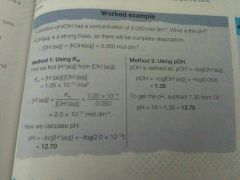Using Kw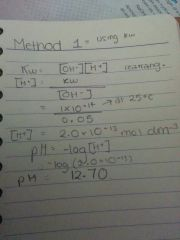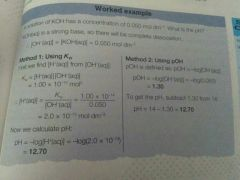Using pOH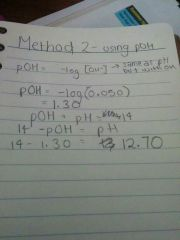Acids, Bases and Buffers MEMORISE at 25 degreesKw = [H+][OH-]and this is all equal to 1.00 x 10 ^-14 mol 2 dm -6. mEMORISE PLS . Acids, Bases and Buffers Define buffer solution A mixture that minimises pH changes on addition of small amounts of acid or base Acids, Bases and Buffers What does a buffer solution consist of? A mixture of a weak acid, HA and its conjugate base, A- Acids, Bases and Buffers How do you make a buffer solution? From a weak acid and salt of the weak acid - for example ethanoic acid + sodium ethanoate Acids, Bases and Buffers How else can you make a buffer solution? By partial neutralisation with an aqueous alkali to give a solution with a mixture of the salt and the excess of weak acid. Acids, Bases and Buffers Explain the buffer solution formed from CH3COOH and CH3COONa. See pic. Acids, Bases and Buffers How does the buffer solution minimise pH change? By using the equilibrium: HA <-> H+ + A-The weak acid, HA removes added alkaliThe conjugate base, A- removes added acid. Acids, Bases and Buffers What happens when you add H+ to a buffer solution? [H+] is increased. Equilibrium shifts to the left, conjugate base reacts with H+ ions, removing them. Acids, Bases and Buffers What happens when OH- (alkali) is added to a buffer solution? [OH-] increased. The small [H+] reacts with the OH- ions H + +OH- ---> H2OThis decreases the [H+] conc, shifting the equilibrium to the right to restore H+ ions that have reacted.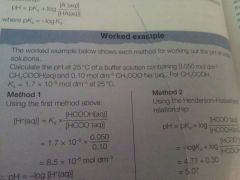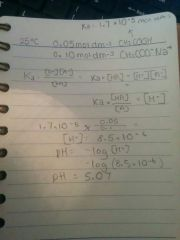Acids, Bases and Buffers Explain the carbonic acid-hydrogencarbonate buffer system. The carbonic acid-hydrogencarbonate ion buffer is the most important buffer system in human blood. H2CO3 (carbonic acid) = weak acid.Hydrogencarbonate, HCO3- = conjugate base. H2CO3 <-> HCO3 - + H+ Increase in H+ in blood = removed by HCO3-. There is a shift in equilibrium to the left, removing H+. Increase in OH- is removed by weak acid, H2CO3. The small conc of H+ ions react with the OH-: H+ + OH- ---> H2O. Then the acid, H2CO3 dissociates, shifting equilibrium to the left to restore most of the H+ ions. Acids, Bases and Buffers What is Ka for this system? 4.3 x 10^-7 mol dm-3. Acids, Bases and Buffers Why is this buffer important? Human blood plasma needs to have a pH between 7.35 and 7.45 Below = acidosisAbove = alkalosis Acids, Bases and Buffers What happens to the carbonic acid produced? It is converted to aqueous CO2 by an enzyme. In the lungs, dissolved CO2 (aq) is converted to CO2 (g) which is then exhaled. CO2 (aq) <-> CO2 (g) Acids, Bases and Buffers What is the equivalence point? The point in a titration at which the volume of one solution has reacted exactly with the volume of the second solution Acids, Bases and Buffers When the acid is in the conical flask and the base in the burette, what pH changes take place? (strong acid - strong base)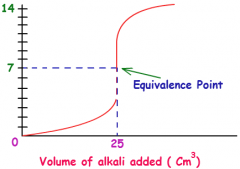- When the base is first added, the pH increases slightly. The acid is in great excess. -Within 1-2cm-3 of the equivalence point, the pH starts to increase more quickly. There is now only a small excess of acid present. Eventually there is a very sharp increase in pH brought about by a very small addition of base, typically just one drop. This is the vertical section on the curve. The equivalence point is the middle of this vertical section.- As further base is added, there is little additional change in pH. The Base is now in great excess. Acids, Bases and Buffers What is an acid-base indicator? Write an equation for methyl orange. A weak acid, represented as HIn. An indicator has one colour in its acid form (HIn) and another in its conjugate base form (In-)For methyl orange: HIn <-> H + + In - Red Yellow Acids, Bases and Buffers Define endpoint. The point in a titration at which there are equal concentrations of the weal acid and conjugate base forms of the indicator.[HIn] = [In-] Acids, Bases and Buffers What is the colour end point for methyl orange? orange, when Hin red and In- yellow are equal. Acids, Bases and Buffers What determines what indicator you use for a titration? An indicator is chosen so that the pH value of the end point is as close to the pH value of of the titration's equivalence point.A suitable indicator changes colour within the pH range in the vertical section of the titration curve.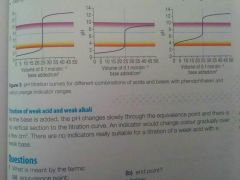Acids, Bases and Buffers Purple = phenolphthaleinOrgane = methyl orange Describe which ones are suitable. a) P - suitableM - suitableb) P - unsuitableM - suitablec) P- suitableM- unsuitable. Acids, Bases and Buffers For titrations that involve weak acids and weak alkalis, describe the titration curve and suitable indicators. As the base is added, the pH changes slowly through the equivalence point and there is no vertical section to the titration curve. An indicator would change colour gradually over a few cm-3, so no indicators are suitable for weak acid- weak base. Acids, Bases and Buffers What happens when an aqueous acid is neutralised by an aqueous base? A salt and water are formed. Acids, Bases and Buffers Define standard enthalpy change of neutralisation. The energy change that accompanies the neutralisation of an aqueous acid by an aqueous base to form one mole of H2O (l) under standard conditions. Acids, Bases and Buffers Draw the full equation, ions and ionic equation for the neutralisation of HCl by NaOH. HCl (aq) + NaOH (aq) ----> NaCl (s) + H2O (l) acid + Base -------> 1 mol ^H*neut = -57.9 kJ mol-1ions: H+ +Cl- + Na+ + OH - ---> Na+ + Cl- + H2O (cancel)ionic equation: H+ + OH- ---> H2O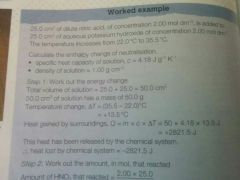Acids, Bases and Buffers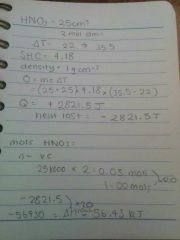pic